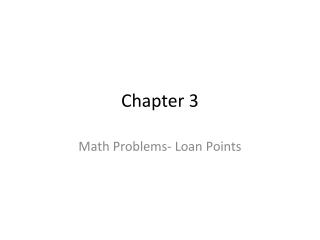# Chapter 3 - PowerPoint PPT PresentationDownload PresentationChapter 3

Chapter 3
Download Presentation## Chapter 3

- - - - - - - - - - - - - - - - - - - - - - - - - - - E N D - - - - - - - - - - - - - - - - - - - - - - - - - - -
##### Presentation Transcript

1. Chapter 3 Math Problems- Loan Points

2. Cash value of Points • You get a loan for \$36,000 at 9% and the lender is charging 1 point. 36,000 x .01 = \$360 (cash value of 1 point) • You get the same loan and the lender is charging 3 points. 36,000 x .03 = \$1080 (cash value of 3 points)

3. Cash value of points on a sliding scale loan • Sales price of a house is \$142,000. The bank will lend 97% on the first \$25,000 and 95% of the balance at 8.5% interest. Origination fee is 3 ½ points. What is the cash value of the points?

4. Solution 1. find the amount over 25,000 \$142,000 - \$25,000 = \$117,000 2. Find the loan amount 97% of \$25,000 = \$24,250 95% of \$117,000 = \$111,150 Total loan amount = \$135,400 3. \$135,400 x .035 = \$4,739 (cash value of 3 ½ pts)

5. Point value to increase lender’s return • The bank loans \$48,000 at 9% plus 5 points. What is the lender’s adjusted rate of interest including the points?

6. Solution • Multiply the 5 points by 1/8 % increase in interest. 5 x 1/8 % = 5/8% • Add the increase return of 5/8 to 9% interest • The adjusted rate of return is 9 5/8% * 1/8 = .125

7. Problem • You purchase a home for \$152,000. The bank lends 80% at 8 ¾% plus 6 points. What is the cash value of the points?

8. Solution • \$152,000 x 80% = \$121,600 \$121,600 x .06 = \$7296 cash value of points • What is the adjusted yield? .125 x 6 points = .75 Add to 8 ¾ % = 9.5 % is the true yield

9. PITI payments Monthly principle and interest is \$826.42. Annual property taxes are \$993.24, annual insurance is \$244.32. What is the monthly PITI payment

10. Solution to PITI Annual taxes: 993.24 /12 = \$82.77 monthly Annual insurance: \$244.32 /12= \$12.36 monthly PI= 826.42 Taxes = 82.77 Ins = 20.36 Total monthly PITI = \$929.55